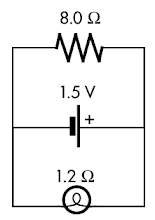## 20140405

### Physics quiz question: current flowing through parallel branch of circuit

Physics 205B Quiz 4, spring semester 2014
Cuesta College, San Luis Obispo, CA

Cf. Giambattista/Richardson/Richardson, Physics, 2/e, Problem 18.40(b)An ideal emf source is connected to a light bulb and a resistor, as shown at right. The current flowing through the light bulb is:
(A) 0.16 A.
(B) 0.19 A.
(C) 1.3 A.
(D) 1.4 A.

Correct answer (highlight to unhide): (C)

From applying Kirchhoff's loop rule in the clockwise direction around the lower loop of the circuit, the voltage rise due to the emf (ε = 1.5 V) must equal the voltage drop due to the 1.2 Ω light bulb (I·R):

ε = I·R,

such that:

I = ε/R = (1.5 V)/(1.2 Ω) = 1.25 A,

or to two significant figures, the current flowing through the 1.2 Ω light bulb is 1.3 A. (Note that this would be less than the current flowing through the emf source, which from Kirchhoff's junction rule would be the sum of the current flowing through the 1.2 Ω light bulb and the current flowing through the 8.0 Ω resistor.)

(Response (A) is 1.5 V divided by (8.0 Ω + 1.2 Ω); response (B) is the current flowing through the 8.0 Ω resistor; and response (D) is the current flowing through the emf.)

Sections 30882, 30883
Exam code: quiz04mCnC
(A) : 3 students
(B) : 2 students
(C) : 20 students
(D) : 11 students

Success level: 56%
Discrimination index (Aubrecht & Aubrecht, 1983): 0.31